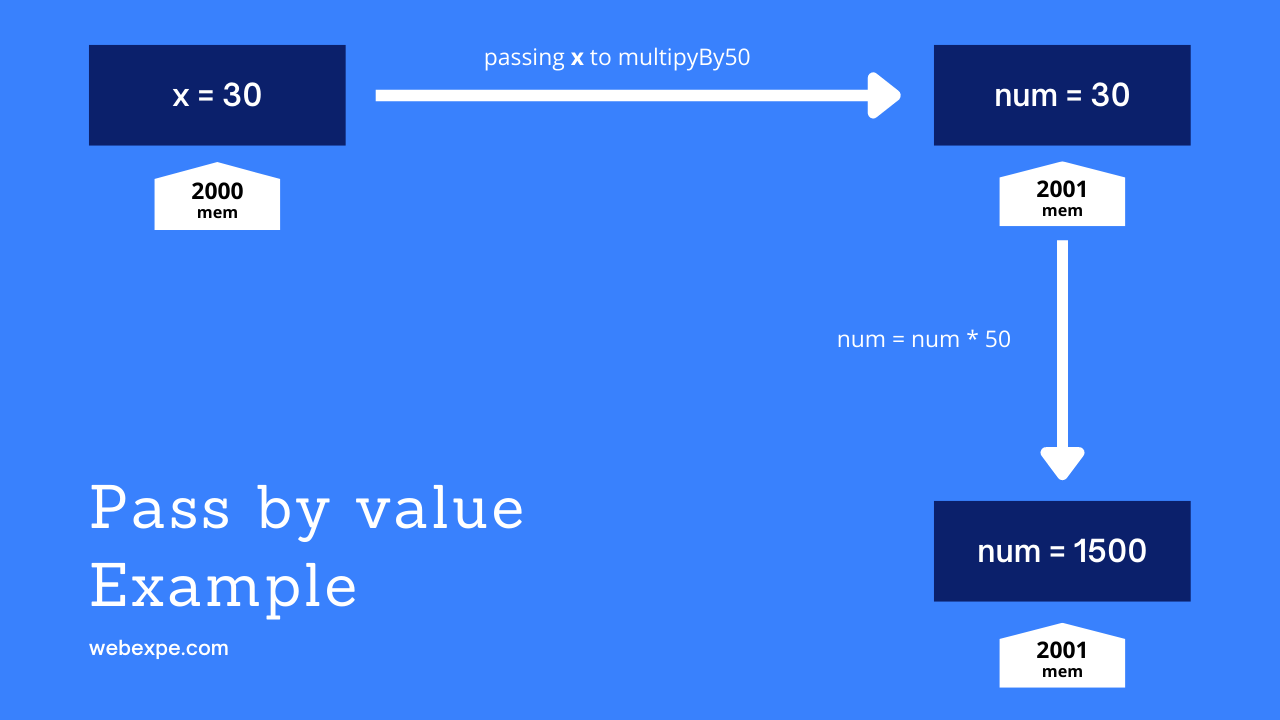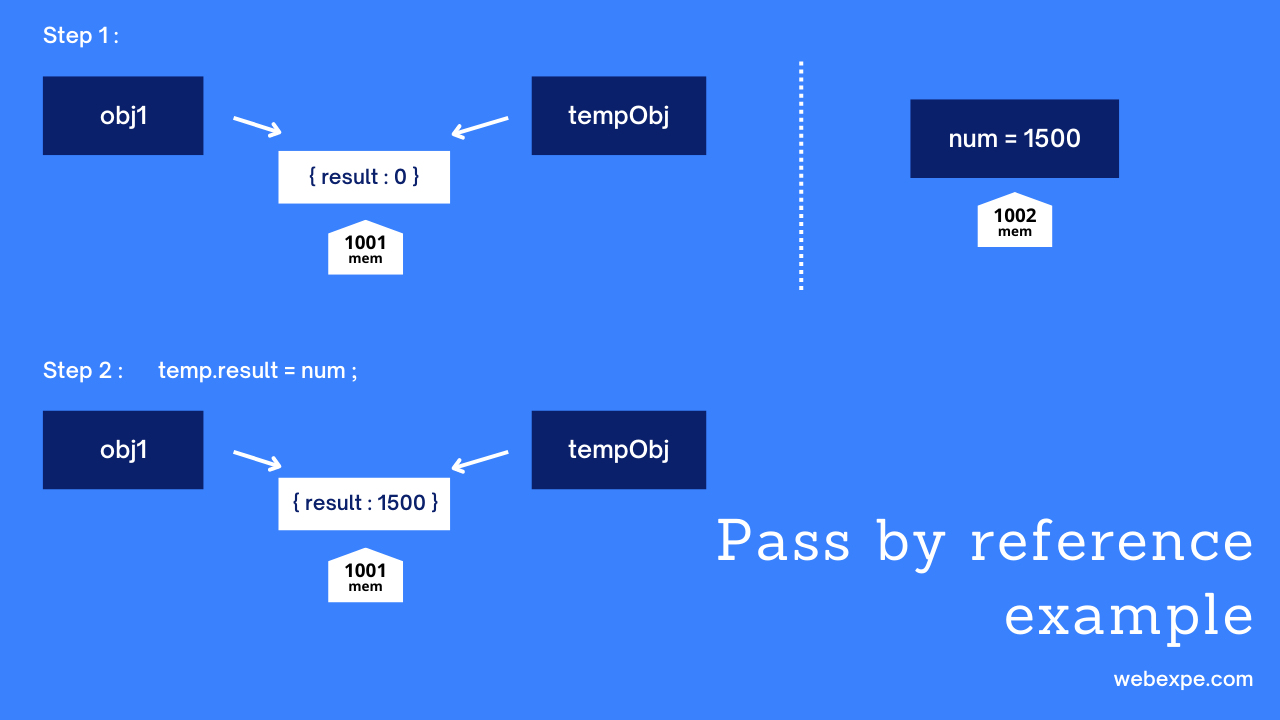# Pass by value and pass by reference | Javascript interview question.

In this article we are going to see difference between Pass by Value and Pass by Reference in JavaScript. Let's dive right in.

## Pass by Value in JavaScript

In Pass by value, a copy of the actual parameter’s value is made in memory. Same addresses are never shared here i.e. all the variables will have different memory addresses with same value.

``````const x = 30;
const multiplyBy50 = (num) => {
num = num * 50;
return num;
};

const ans1 = multiplyBy50(x);``````In the above code snippet, we see a const x with value 30 is created with an address 2000. This x is passed to multiplyBy50 function. Javascript automatically copies the value of x to variable num i.e. a new address was created with a copy of x's value. when num is reassigned the product of num and 50 the value of x doesn't change as address of x and num are different. This is pass by value.

## Pass by Reference in JavaScriptIn Pass by Reference, the address/reference of the variable is passed to the function. Let's look at the example below to understand this better. Two parameters are passed to function multiplyBy50 i.e. x and ogObj. Executing statement tempObj.result = num; will change the result property of ogObj to 1500 from 0 because in javascript, a reference to an object is passed to a function by value i.e. address of ogObj was passed instead of its value.

``````    const x = 30;
const ogObj = { result: 0 };

const multiplyBy50 = (num, tempObj) => {
num = num * 50;
tempObj.result = num;
console.log(tempObj);  // { result: 1500 };
return num;
}

const ans1 = multiplyBy50(x, ogObj);``````

In conclusion, Everything in JavaScript is of value type and function arguments are always passed by value but with objects this turns out a little differently 😜.

passByValue
passByReference
javaScript
style with uncontrolled components